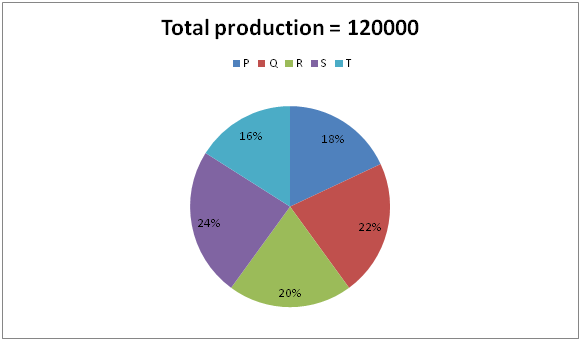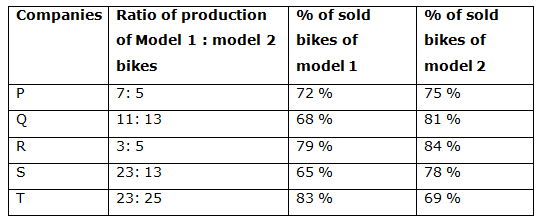# IBPS Clerk Mains Quantitative Aptitude Questions 2019 – (Day-12)

Dear Aspirants, Our IBPS Guide team is providing new pattern Quantitative Aptitude Questions for IBPS Clerk Mains 2019 so the aspirants can practice it on a daily basis. These questions are framed by our skilled experts after understanding your needs thoroughly. Aspirants can practice these new series questions daily to familiarize with the exact exam pattern and make your preparation effective.

[WpProQuiz 7538]

Data Interpretation

Directions (Q. 1 – 5): Study the following information carefully and answer the given questions?

The following pie chart shows the total production of bikes of 5 different companies and the table shows the total number of model 1 and model 2 bikes produced by them and the percentage distribution of bikes sold by them.1) Find the difference between the total production of model 1 bikes in company P, R and T together to that of total production of model 2 bikes in company Q, S and T together?

a) 4000

b) 3600

c) 3900

d) 4200

e) None of these

2) Find the total number of model 1 bikes sold by company P, Q and R together?

a) 24410

b) 25680

c) 23850

d) 26540

e) None of these

3) Find the ratio between the total number of bikes produced by company P, R and S together to that of total number of model 2 bikes produced by company S and T together?

a) 54: 19

b) 45: 23

c) 72: 35

d) 62: 17

e) None of these

4) Total number of model 2 bikes sold by company P and R together is approximately what percentage of total number of model 1 bikes produced by company Q and R together?

a) 108 %

b) 92 %

c) 80 %

d) 65 %

e) 120 %

5) Find the difference between the average number of model 1 bikes sold by company Q and T together to that of the average number of model 1 bikes sold by company S and T together?

a) 2170

b) 2356

c) 1866

d) 2412

e) None of these

Application sums

6) Rahul borrowed a total amount of Rs. 60000, a part of it at simple interest of 12% per annum and the remaining at 10% per annum. If at the end of 3 years, he paid Rs. 79500 to settle the whole loan amount, and then find the amount borrowed at 10% per annum?

a) Rs. 30000

b) Rs. 35000

c) Rs. 32000

d) Rs. 28000

e) None of these

7) Rajeev, Mano and Kalai enter into a partnership by investing in the ratio of (3/7) : (5/3) : (7/6). After 6 months, Rajeev increased his investment by 50 %. If the total profit at the end of the year is Rs. 169800, then find the share of Kalai?

a) Rs. 69200

b) Rs. 58800

c) Rs. 72500

d) Rs. 64600

e) None of these

8) 5years ago, the age of the father and son is in the ratio of 7: 2. Eight years after, the age of the father and 5 years after the age of the son is in the ratio of 12 : 5. The average Present age of the father, mother, son and daughter is 25 years. The difference between the age of the mother and her daughter is 25 years. Then find the present age of daughter?

a) 10 years

b) 18 years

c) 14 years

d) 16 years

e) None of these

9) A and B together can complete the job in 20 days, B and C together can complete the job in 24 days. First A did the job for 8 days, B did the job for 14 days and C completed the remaining job in 20 days. Find the number of days in which C alone can complete the job?

a) 40 days

b) 48 days

c) 30 days

d) 36 days

e) None of these

10) Manju spent 20 % of his monthly salary on food, 18 % on children’s education, 12 % on insurance and X % on other expenses. If the difference between the amount spent on children’s education and insurance is Rs. 4500 and the saving is Rs. 22500, then find the value of X?

a) 25 %

b) 20 %

c) 35 %

d) 30 %

e) None of these

Directions (1-5) :

The total production of model 1 bikes in company P, R and T together

= >120000 * (18/100) * (7/12) + 120000 * (20/100) * (3/8) + 120000 * (16/100) * (23/48)

= >12600 + 9000 + 9200 = 30800

The total production of model 2 bikes in company Q, S and T together

= >120000 * (22/100) * (13/24) + 120000 * (24/100) * (13/36) + 120000 * (16/100) * (25/48)

= >14300 + 10400 + 10000 = 34700

Required difference = 34700 – 30800 = 3900

The total number of model 1 bikes sold by company P, Q and R together

= > 120000 * (18/100) * (7/12) * (72/100) + 120000 * (22/100) * (11/24) * (68/100) + 120000 * (20/100) * (3/8) * (79/100)

= >9072 + 8228 + 7110 = 24410

The total number of bikes produced by company P, R and S together

= >120000 * (62/100) = 74400

The total number of model 2 produced sold by company S and T together

= >120000 * (24/100) * (13/36) + 120000 * (16/100) * (25/48)

= > 10400 + 10000 = 20400

Required ratio = 74400: 20400 = 62: 17

Total number of model 2 bikes sold by company P and R together

= > 120000 * (18/100) * (5/12) * (75/100) + 120000 * (20/100) * (5/8) * (84/100)

= > 6750 + 12600 = 19350

Total number of model 1 bikes produced by company Q and R together

= > 120000 * (22/100) * (11/24) + 120000 * (20/100) * (3/8)

= > 12100 + 9000 = 21100

Required % = (19350 / 21100) * 100 = 92 %

The average number of model 2 bikes sold by company Q and T together

= >[120000 * (22/100) * (11/24) * (68/100) + 120000 * (16/100) * (23/48) * (83/100)] / 2

= > (8228 + 7636) / 2 = 7932

The average number of model 1 bikes sold by company S and T together

= >[120000 * (24/100) * (23/36) * (65/100) + 120000 * (16/100) * (23/48) * (83/100)] / 2

= > (11960 + 7636) / 2 = 9798

Required difference = 9798 – 7564 = 1866

Directions (6-10) :

According to the question,

= > [(x* 12 * 3)/100] + [((60000 – x)* 10 * 3)/100] = 19500

= > (36x/100) + 18000 – (30x/100) = 19500

= > 18000 + (6x/100) = 19500

= > (6x/100) = 19500 – 18000

= > (6x/100) = 1500

= > x = 25000

The amount borrowed at 10 % per annum = 60000 – x = Rs. 35000

Rajeev, Mano and Kalai enter into a partnership by investing in the ratio

= > (3/7) : (5/3) : (7/6).

= >18 : 70 : 49

The shares of Rajeev, Mano and Kalai

= > [18 * 6 + 18 *(150/100)* 6] : [70 * 12] : [49 *12]

= >270 : 840 : 588

= >45 : 140 : 98

Total profit = 169800

283’s = 169800

1’s = 600

The share of Kalai = 98 * 600 = Rs. 58800

5 years ago, the ratio of age of the father and his son = 7: 2 (7x, 2x)

8 years after, the ratio of age of the father and 5 years after, the age of son = 12: 5

According to the question,

(7x + 13)/(2x + 10) = (12/5)

35x + 65 = 24x + 120

11x = 55

x = 5

The present age of the father and his son = (7x + 5), (2x + 5) = 40, 15

The average Present age of the father, mother, son and daughter = 25

Total Present age of the father, mother, son and daughter = 25 * 4 = 100

Total present age of the mother and her daughter = 100 – 55 = 45

Let the present age of mother and daughter be A and B,

A + B = 45 —> (1)

A – B = 25 —> (2)

By solving the equation (1) and (2),

A = 35, B = 10

The present age of daughter = 10 years

LCM of 20 and 24 = 120 units

(A + B)’s one day work = 6 units

(B + C)’s one day work = 5 units

A’s 8days work + B’s 14days work + C’s 20 days work = 120

(A + B)’s 8 days work + (B + C)’s 6days work + C’s 14 days work = 120

(8 * 6) + (5 * 6) + C’s 14 days work = 120

C’s 14 days work = 120 – 48 – 30 = 42

C’s one day work = 42/14 = 3 units

C alone can complete the work in, (120/3) = 40 days

According to the question,

(18 % – 12 %) of salary = 4500

6 % of salary = 4500

Total salary = 4500* (100/6) = Rs. 75000 = 100 %

Savings % = (22500/75000)* 100 = 30 %

Total salary (100 %) = Expense (70 %) + Savings (30 %)

Given,

Expense = 70 %

70 % = (20 % + 18 % + 12 % + X %)

X % = 70 % – 50 % = 20 %